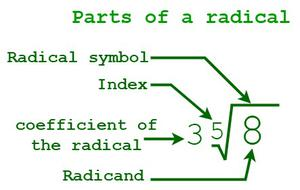GFG App
Open AppBrowser
ContinueIn the figure given above, “3” is the co-efficient of the radical, “5” is the index, “8” is the radicand, and “(√)” is the radical symbol.

### Some rules for solving Radicals

Product Rule: If “(n√a)” and  “(n√b)”are real numbers and n is a natural number, then

n√(a × b) = n√a ×  n√b, where a, b ∈ R, n ∈ N.

Quotient rule: If “(n√a)” and  “(n√b)”are real numbers and n is a natural number, then

n√(a /b) = n√a /n√b,  where a, b ∈ R, n ∈ N, b≠0

Fractional exponent: If “(n√a)” is a real number, n is a natural number and m is an integer, then,

n√(am) = a(m/n)

Let us consider another example to understand the concept better.

Example 1: Solve: [3√2 + 7√2].

Solution:

Here,

That means we can add both terms.

So,

[3√2 + 7√2] = (3 + 7)√2

= 10√2.

Example 2: Solve 2√27 + 6√16 – 5√3.

Solution:

The given expression is 2√27 + 6√16 – 5√3.

Here, the radicands of the three terms are different. But the radicands can be simplified further.

2√(33) + 6√(42) + 5√3 = 2 × 3√3 + 6 × 4 – 5√3

= 6√3 + 24 – 5√3

= 24 + (6 – 5)√3 = 24 + √3.

Hence,

2√27 + 6√16 – 5√3 = 24 + √3.

• First, break down the given radicals and then simplify each term.

Example: Solve 21√2 + 10√5 −9√2 + 7√5.

Solution:

Given expression: 21√3 + 10√7 −9√3 + 7√7

= (21√3 – 9√3) + (10√7 + 7√7)

Now, add or subtract the coefficients of the like terms.

= 12√3 + 17√7

We cannot simplify any further as √3 and √7  are not like radicals since their radicands are different.

Hence, 21√3 + 10√7 −9√3 + 7√7 = 12√3 + 17√7.

### Sample Problems

Problem 1: Solve 6√27 − 2√12 + √48.

Solution:

Given expression: 6√27 − 2√12 + √48

= 6√(3 × 3 × 3) − 2√(2 × 2 × 3) + √(4 × 4 × 3)

= 6 [√(3×3) × √3] − 2[√(2 × 2) × √3] + √(4 × 4) × √3  {Since, n√(a × b)= n√a × n√b}

= 6 × 3√3 − 2 × 2√3 + 4√3

= 18√3 − 4√3 + 4√3

= 18√3

Thus, 6√27 – 2√12 + √48 = 18√3.

Problem 2: Solve:  2 3√162 − 5 3√40 + 3 3√48 +7 3√135

Solution:

Given expression: 2 3√162 − 5 3√40 + 3 3√48 + 7 3√135

= 2 3√(27 × 6) − 5 3√(8 × 5) + 3 3√(8 × 6) + 7 3√(27 × 5)

= 2 [3√27 × 3√6] – 5[3√8 × 3√5] + 3[3√8 × 3√6] + 7[3√27 × 3√5]  {Since, n√(a × b)= n√a × n√b}

= (2 × 3 × 3√6) − (5 × 2 × 3√5) + (3 × 2 × 3√6) + (7 × 3 × 3√5)

= 6 3√6 − 10 3√5 + 6 3√6 + 21 3√5

= 12 3√6 + 11 3√5

Hence, 2 3√162 − 5 3√40 + 3 3√48 +7 3√135 = 12 3√6 + 11 3√5

Problem 3: Solve: 4√(x6y) + 7√(xy2) − √(y2x).

Solution:

Given expression: 4√(x6y) + 7√(xy2) − √(y2x)

= 4 [√x6 × √y] + 7[√x × √y2] − [√y2 × √x]    {Since, n√(a × b) = n√a × n√b}

= 4 [√(x3)2 × √y] + 7[√x × y] −[√y2 × √x]    {Since, amn = (am)n}

= 4x3√y + 7y√x − y√x

= 4x3√y + 6y√x

Thus, 4√(x6y) + 7√(xy2) − √(y8x) = 4x3√y + 6y√x.

Problem 4: Solve: 13√11 −12√17 − 22√11 + 20√17 + 18√11.

Solution:

Given expression: 13√11 −12√17 − 22√11 + 20√17 + 18√11

= (13√11 – 22√11 + 18√11) + (-12√17 + 20√17)

= (31√11 – 22√11) + (8√17)

= 9√11 + 8√17.

Thus, 13√11 −12√17 − 22√11 + 20√17 + 18√11 = 9√11 + 8√17.

Problem 5: Solve:  2[√a + 5√(3b)]− 8[√a – 11√(3b)] + 14[3√a + 2√(3b)]

Solution:

Given expression: 2[√a + 5√(3b)]− 8[√a – 11√(3b)] + 14[3√a – 2√(3b)]

= 2√a + 10√(3b) -8√a + 88√(3b) + 42√a – 28√(3b)

= [2√a – 8√a + 42√a] + [10√(3b) + 88√(3b) – 28√(3b)]

= 36√a + 70√(3b)

Thus, 2[√a + 5√(3b)]− 8[√a – 11√(3b)] + 14[3√a + 2√(3b)] =  36√a + 70√(3b).

Problem 6: Solve: −11 5√(2xy) + 23 5√(2xy) − 5√(64xy) + 15 5√(2xy).

Solution:

Given expression: −11 5√(2xy) + 23 5√(2xy) − 5√(64xy) + 15 5√(2xy)

= −11 5√(2xy) + 23 5√(2xy) − 5√(32 × 2xy) +15 5√(2xy)

= −11 5√(2xy) − 5√32 × 5√(2xy) + 23 5√(2xy) + 15 5√(2xy)

= −11 5√(2xy) − 2 5√(2xy) + 38 5√(2xy)

= −13 5√(2xy) + 38 5√(2xy)

= 25 5√(2xy)

Hence, −11 5√(2xy) + 23 5√(2xy) − 5√(64xy) + 15 5√(2xy) = 25 5√(2xy)

My Personal Notes arrow_drop_up